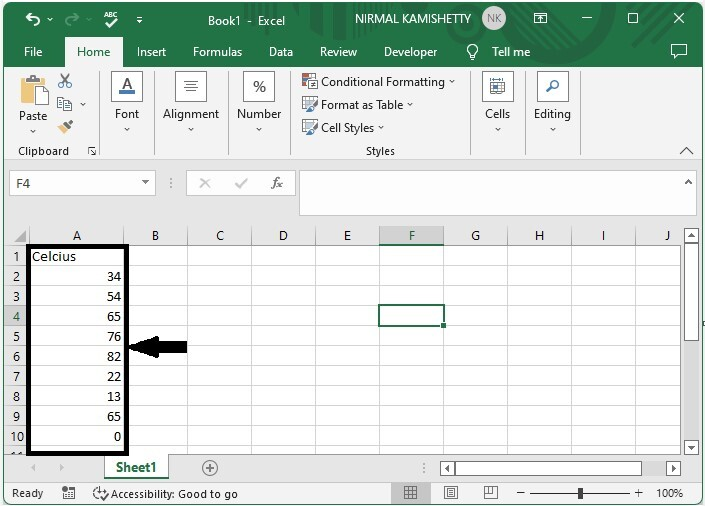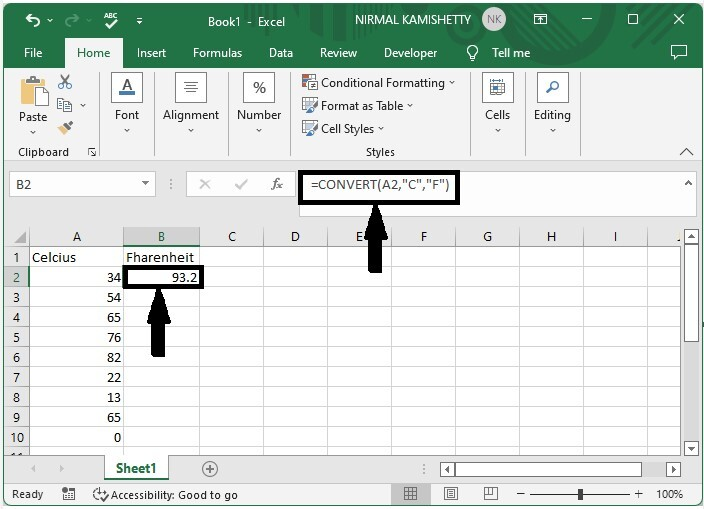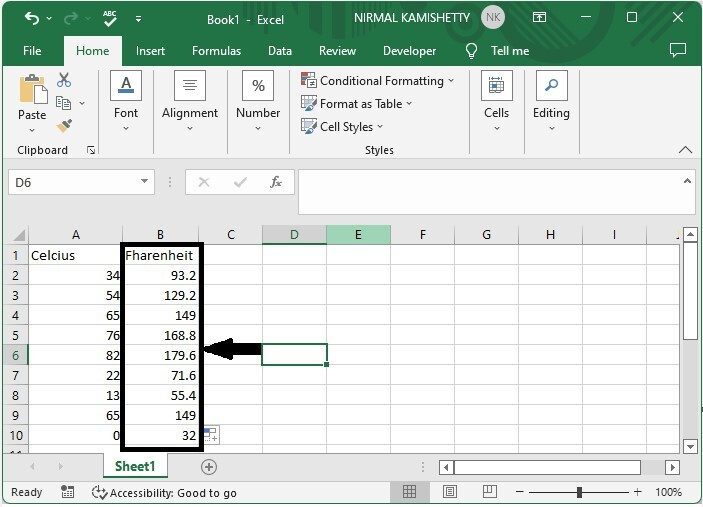# How to Convert Temperature Units between Celsius, Kelvin and Fahrenheit in Excel?

The degree of hotness or coldness of any object is known as its temperature. Generally, temperature can be measured on these scales: Celsius, Kelvin, and Fahrenheit. Different people will measure temperature on different scales. So, changing the scale of temperature can be used frequently when dealing with temperatures.

Read this tutorial to learn how you can convert temperature units between Celsius, Kelvin, and Fahrenheit in Excel, i.e., changing the values from Celsius to kelvin or Fahrenheit and vice versa. We can solve this process by using formulas supported by Excel.

## Converting Temperature Units between Celsius, Kelvin and Fahrenheit in Excel

Here we will use the CONVERT formula to get any one of the results, then use the auto-fill handle to get all the results. Let us see a simple process to know how we can convert temperature units between Celsius, kelvin, and Fahrenheit in Excel.

### Step 1

Let us consider an Excel sheet where we have the list of temperatures in Celsius, similar to the below image.Now to convert the Celsius to Fahrenheit, click on an empty cell and enter the formula as =CONVERT(A2,"C","F") and click enter to get the first result that looks similar to the below image. "C" stands for Celsius and "F" stands for Fahrenheit in the formula A2, where "A" is the address of our value in the Excel sheet.### Step 2

To get all the results, drag from the first result using the auto-fill handle.To convert Celsius to Kelvin, we can use the formula as =CONVERT(A2,"C","K").

Note − We can use the same format formula for all conversions.

## Conclusion

In this tutorial, we used a simple example to demonstrate how you can convert temperature units between Celsius, Fahrenheit, Kelvin in Excel.